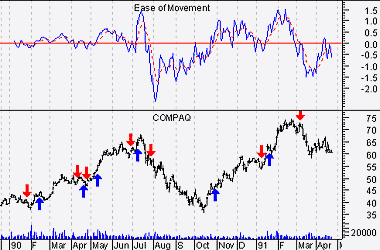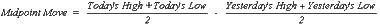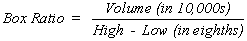# Technical Analysis from A to Z

by Steven B. Achelis

EASE OF MOVEMENT

Overview

The Ease of Movement indicator shows the relationship between volume and price change. As with Equivolume charting, this indicator shows how much volume is required to move prices.

The Ease of Movement indicator was developed Richard W. Arms, Jr., the creator of Equivolume.

Interpretation

High Ease of Movement values occur when prices are moving upward on light volume. Low Ease of Movement values occur when prices are moving downward on light volume. If prices are not moving, or if heavy volume is required to move prices, then the indicator will also be near zero.

The Ease of Movement indicator produces a buy signal when it crosses above zero, indicating that prices are moving upward more easily; a sell signal is given when the indicator crosses below zero, indicating that prices are moving downward more easily.

Example

The following chart shows Compaq and a 14-day Ease of Movement indicator. A 9-day moving average was plotted on the Ease of Movement indicator."Buy" and "sell" arrows were placed on the chart when the moving average crossed zero.

Calculation

To calculate the Ease of Movement indicator, first calculate the Midpoint Move as shown below.Next, calculate the "High-Low" Box Ratio expressed in eighths with the denominator dropped (e.g., 1-1/2 points = 12/8 or just 12).The Ease of Movement ("EMV") indicator is then calculated from the Midpoint Move and Box Ratio.

The raw Ease of Movement value is usually smoothed with a moving average.

This online edition of Technical Analysis from A to Z is reproduced here with permission from the author and publisher.